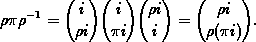Conjugation

### Conjugation

Having described the elements of Sn , we show which of them are in the same conjugacy class, i.e. in the same orbit of the group Sn on the set Sn under the conjugation action. In order to do this, we first note how rpr-1 is obtained from the permutation p:Thus, in terms of cyclic factors of p, rpr-1 arises from
p = ( ...i pi ...) ...
by simply applying r to the points in the cycles of p:
rpr-1= ...( ...ri r( pi) ...) ... .
(For any mapping j:S -> T we mean by j(s)=t that s has to be replaced by t and not that t is replaced by s, as it is sometimes understood!) This equation shows that the lengths of the cyclic factors of p are the same as those of rpr-1. It is easy to see that, conversely, for any two elements p, sÎSn with the same lengths l n of cyclic factors there exists a rÎSn such that rpr-1= s. Hence the lengths of the cyclic factors of p characterize its conjugacy class. To make this more explicit, we introduce the notion of (proper) partition of n Î N, by which we mean any sequence a= ( a1, a2, ...) of natural numbers ai which satisfy
" i : ai >= ai+1, and åi ai=n.
The ai are called the parts of a. The fact that a is a partition of n is abbreviated by
an.
If an then there exists an h such that ai=0 for all i>h. We may therefore write
a=( a1, ..., ah),
for any such h. The minimal h with this property will be denoted by l( a) and called the length of a. The following abbreviation is useful in the case when several nonzero parts of a are equal, say ai parts are equal to i,i În:
a= (nan,(n-1)an-1, ...,1a1).
If ai=0, then iai is usually omitted, e.g. (3,12)=(3,1,1,0, ...). For pÎSn the ordered lengths ai( p),i Î c( p), of the cyclic factors of p in cycle notation form a uniquely determined proper partition
a( p)=( a1( p), a2( p), ..., ac( p) ( p)) n,
which we call the cycle partition of p. The corresponding n-tuple
a( p):=(a1( p), ...,an( p))
consisting of the multiplicities ai( p) of the parts of length i in a( p) is called the cycle type of p. Correspondingly we call an n-tuple a:=(a1, ...,an) a cycle type of n if and only if each ai Î N, and åi ·ai =n. This will be abbreviated by
a |¾| n.

harald.fripertinger@kfunigraz.ac.at,
last changed: August 28, 2001Conjugation